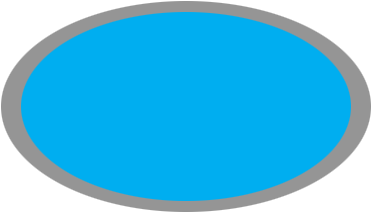SEARCH HOMEMath Central Quandaries & QueriesQuestion from steve: I have a 16' x 28' oval pool that is buried 24" deep inground. The dig site is dug 2' wider all the way around the pool. I need to back fill this area with stone. I want to fill this area with 6 to 8" of stone. How many tons of stone will this take? Thanks you SteveHi Steve,

An oval is not well defined term so I am going to assume the shape is an ellipse.The area of an ellipse is given by $A = \pi \; a b$ where $a$ and $b$ are half the lengths of the major and minor axes. The ellipse formed by the pool and the band around the pool is 20' by 32' and hence the area of this ellipse is $\pi \; \times 10 \times 16$ square feet. The area of the pool itself is $\pi \; \times 8 \times 14$ square feet. Hence the area of the band around the pool is

$\pi \; \times 10 \times 16 - \pi \; \times 8 \times 14 = 150.80 \mbox{ square feet.}$

The depth of the stone is 8" and hence its volume is $150.80 \times \large \frac{8}{12} \normalsize = 100.53$ cubic feet. There are 3 feet in a yard so the volume of stone needed is

$\frac{100.53}{3 \times 3 \times 3} = \frac{100.53}{27} = 3.7 \mbox{ cubic yards.}$

The weight of the stones depends on the type of stone you are using. I suggest that you tell your contractor that you need 3 1/2 cubic yards.

PennyMath Central is supported by the University of Regina and the Imperial Oil Foundation.